# 18.33. Usar lineas de codigo R en Processing¶

Module contributed by Matteo Ghetta - funded by Scuola Superiore Sant’Anna

Processing (with the `Processing R Provider` plugin) makes it possible to write and run R scripts inside QGIS.

R has to be installed on your computer and the PATH has to be correctly set up. Moreover Processing just calls the external R packages, it is not able to install them. So be sure to install external packages directly in R. See the related chapter in the user manual.

Nota

If you have package problems, it may be related to missing mandatory packages required by Processing, like `sp`, `rgdal` and `raster`.

## 18.33.1. Agregar lineas de codigo¶

Adding a script is simple. The easiest way is to open the Processing toolbox and choose Create new R script… from the R menu (labelled with an R icon) at the top of the Processing Toolbox. You can also create the script in for instance a text editor and save it in your R scripts folder (`processing/rscripts`). When it has been saved there, it will be available for editing by right-clicking on the script name in the processing toolbox and then choose Edit Script…).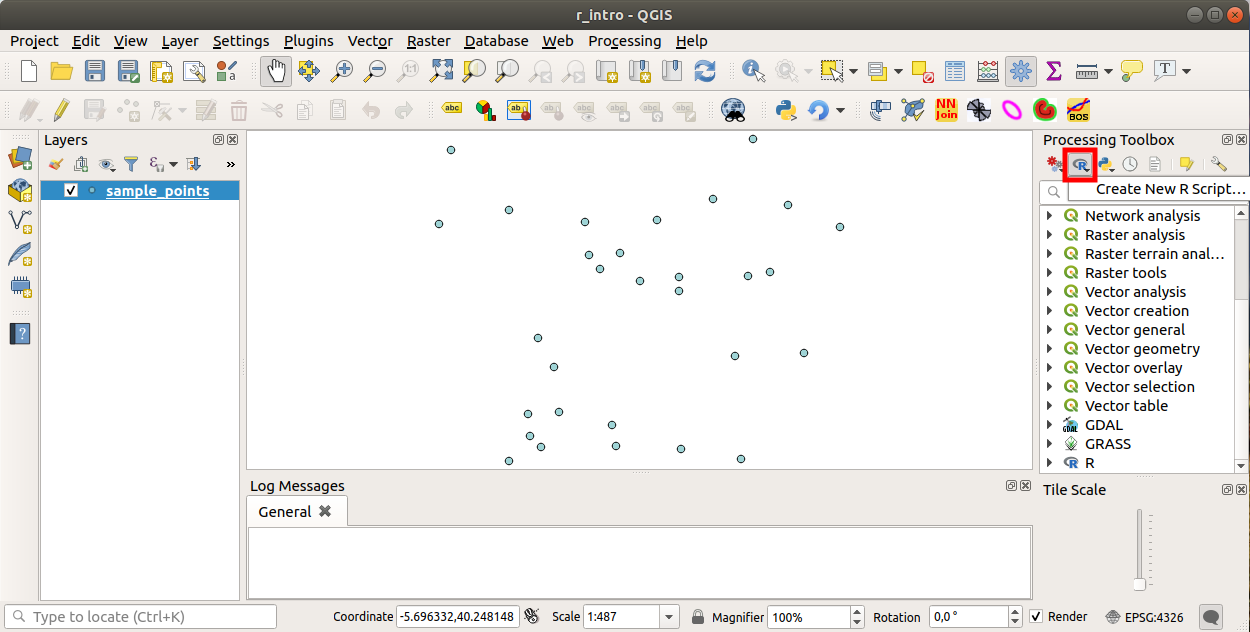Nota

Si no puedes ver R en Processinf, tienes que activarlo en Processing ‣ Options ‣ Providers

Abre una «ventana de edicion de lineas de codigo» en el cual tienes que especificar algunos parametros antes de agregar el codigo.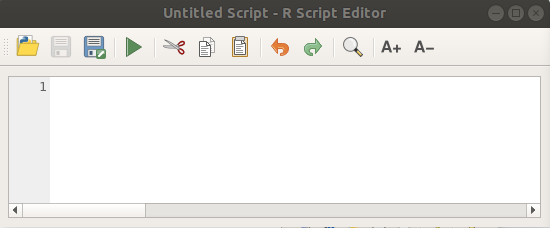## 18.33.2. Crear parcelas¶

En este tutorial vamos a crear una «caja de parcelas» de un campo de capas vectoreales.

Abra el proyecto QGIS `r_intro.qgs` que esta en la carpeta `exercise_data/processing/r_intro/`.

### 18.33.2.1. Parametros de linea de codigo¶

Abrir el editor y comenzar a escribir al inicio.

«Tienes» que especofocar algunso parametros «antes» del cuerpo de codigo de lineas;

1. The name of the group (plots in this case) in which you want to put your script (if the group does not exist, it will be created):

```##plots=group
```

You will find your script in the plots R group in the Processing toolbox.

2. You have to tell Processing that you want to display a plot (in this example):

```##showplots
```

You will then find a link to the plot in the Result Viewer panel (can be turned on / off in View ‣ Panels and with Processing ‣ Results Viewer).

3. You also need to tell Processing about your input data. In this example we want to create a plot from a field of a vector layer:

```##Layer=vector
```

Processing now knows that the input is a vector. The name Layer is not important, what matters is the vector parameter.

4. Finally, you have to specify the input field of the vector layer (using the name you have provided above - Layer):

```##X=Field Layer
```

Processing now knows that you need a field of Layer, and that you will call it X.

5. It is also possible to define the name of your script using `name`:

```##My box plot script=name
```

If not defined, the file name will be used as the name of the script.

### 18.33.2.2. Cuerpo de lineas de codigo¶

Ahora que tienes la «cabecera» de las lineas de codigo puedes agregar las funciones;

```boxplot(Layer[[X]])
```

boxplot is the name of the R function, the parameter Layer is the name that you have defined for the input dataset and X is the name you have defined for the field of that dataset.

The parameter X has to be within double square brackets (`[[]]`).

El codigo final se ve asi:

```##Vector processing=group
##showplots
##Layer=vector
##X=Field Layer
boxplot(Layer[[X]])
```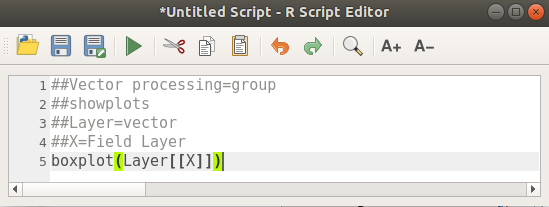Save the script in the default path suggested by Processing (processing/rscripts). If you have not defined a `name` in the script heading, the file name you choose will become the name of the script in the Processing toolbox.

Nota

You can save the script wherever you like, but Processing will then not be able to include it in the processing toolbox automatically, so you have to upload it manually.

Ahora puedes correrlo usando el botón en la parte superior de la ventana de edición: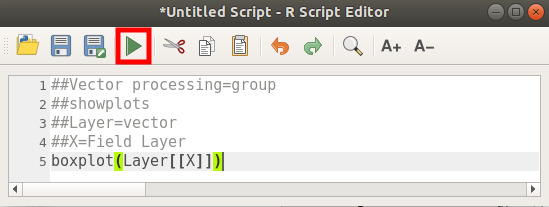Once the editor window has been closed, use the text box of Processing to find your script: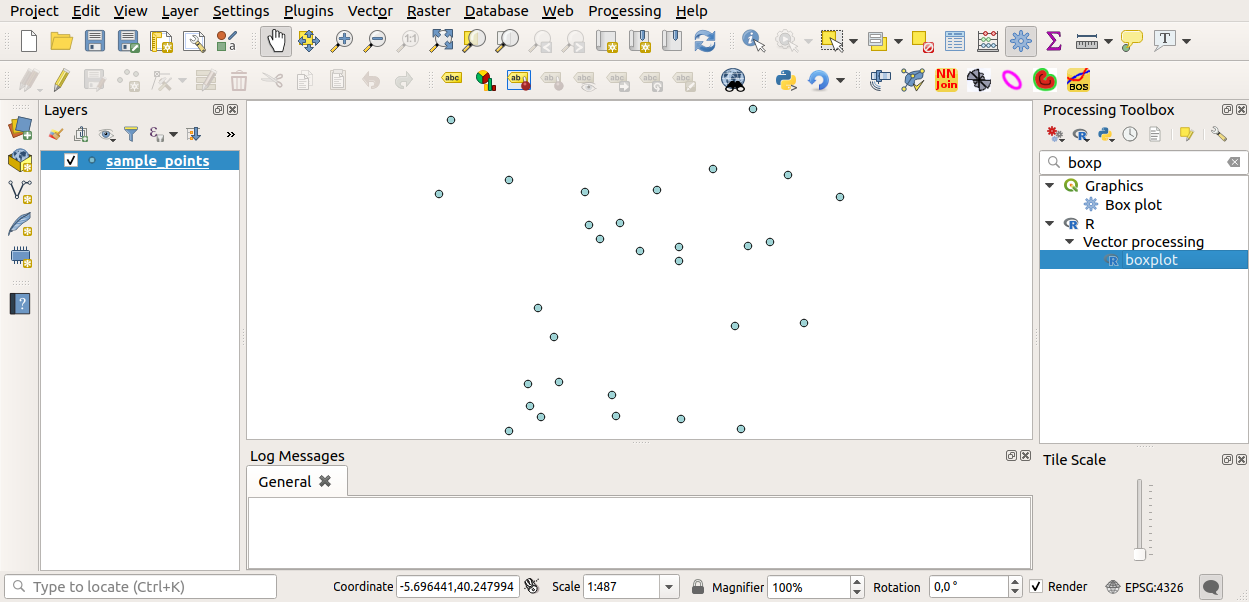You can now fill the parameters required in the Processing algorithm window:

• for Layer choose sample_points

• for the X field choose value

Elegir «Run»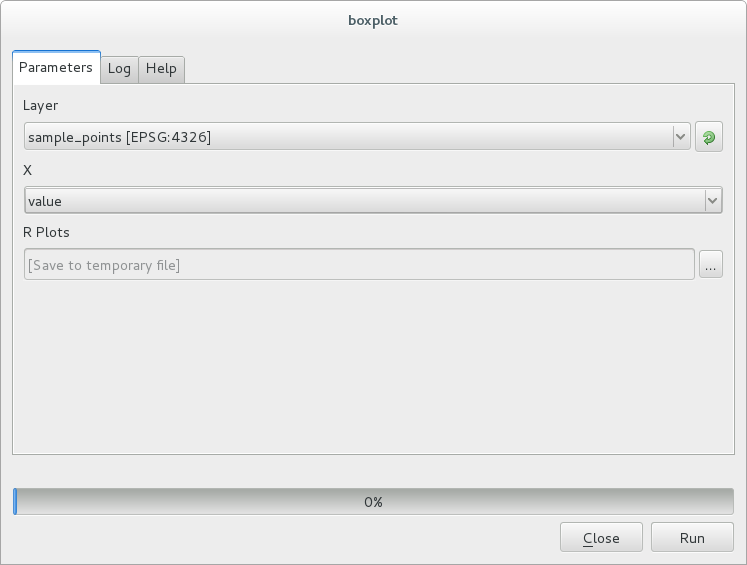La «Ventana de Resultados» se debe abrir automaticamente, si no, solo hacer click en Processing ‣ Result Viewer….

Click on the link in the viewer and you will see: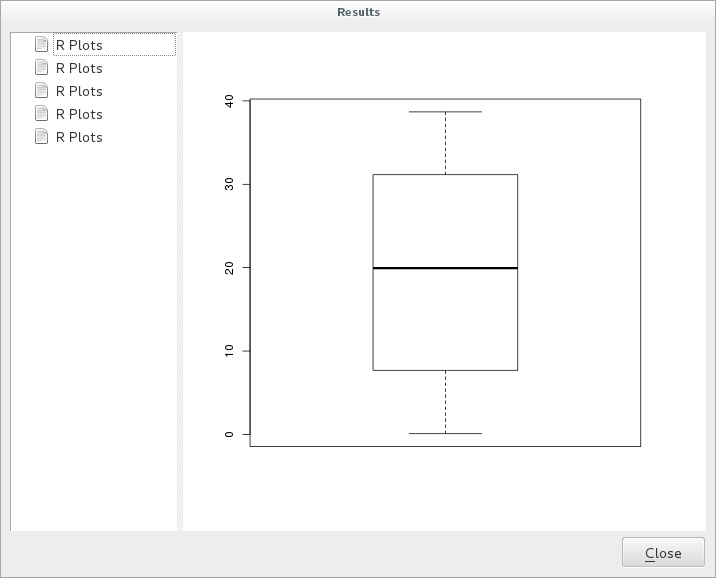Nota

You can open, copy and save the image by right clicking on the plot.

## 18.33.3. Crear un vector¶

You can also create a vector layer and have it automatically loaded into QGIS.

The following example has been taken from the `Random sampling grid` script that you can download from the online collection R ‣ Tools ‣ Download R scripts from the on-line collection (the scripts in the on-line collection can be found on https://github.com/qgis/QGIS-Processing/tree/master/rscripts).

The aim of this exercise is to create a random point vector layer using an input vector layer to restrict the extent using the `spsample` function of the `sp` package.

### 18.33.3.1. Parametros de linea de codigo¶

Igual que aneriormente hay que poner parametros antes del cuerpo del codigo:

1. Specify the name of the group in which you want to put your script, in this case Point pattern analysis:

```##Point pattern analysis=group
```
2. Define an input parameter (a vector layer) that will contstrain the placement of the random points:

```##Layer=vector
```
3. Set an input parameter for the number of points that are going to be created (`Size`, with a default value of `10`):

```##Size=number 10
```

Nota

Since a default value (`10`) is defined, the user can change this number or can leave the parameter without a number.

4. Specify that there is an output vector layer (called `Output`):

```##Output=output vector
```

### 18.33.3.2. Cuerpo de lineas de codigo¶

Ahora que puedes agregar el cuerpo de la funcion:

1. Use the `spsample` function:

```pts=spsample(Layer, Size, type="random")
```

The function uses the Layer to constrain the placement of the points (if it is a line layer, a points will have to be on one of the lines in the layer, if it is a polygon layer, a point will have to be within a polygon). The number of points is taken from the Size parameter. The sampling method is random.

2. Generate the output (the `Output` parameter):

```Output=SpatialPointsDataFrame(pts, as.data.frame(pts))
```

El codigo final se ve asi:

```##Point pattern analysis=group
##Layer=vector
##Size=number 10
##Output=output vector
pts=spsample(Layer, Size, type="random")
Output=SpatialPointsDataFrame(pts, as.data.frame(pts))
```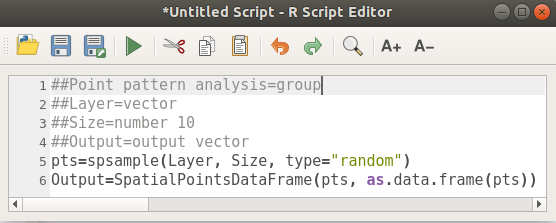Save it and run it, clicking on the run button.

En la ventana nueva escriba los parámetros correctos: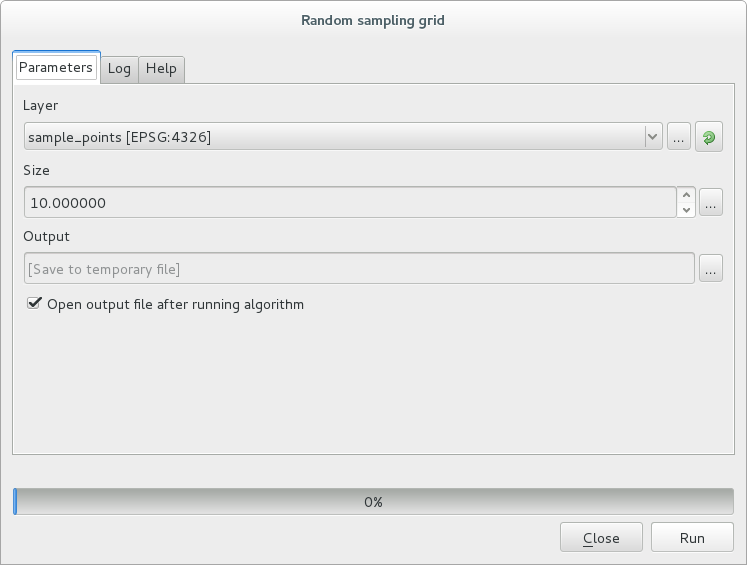haga click en ejecutar.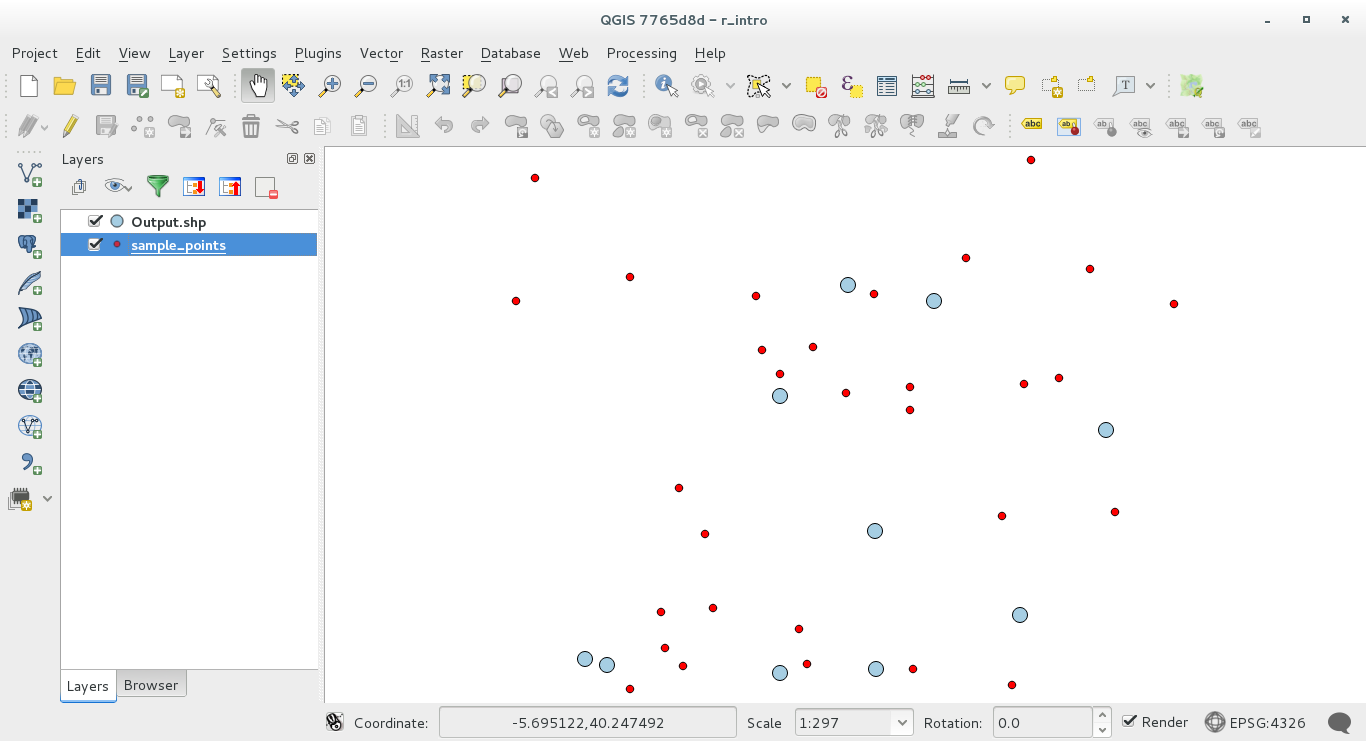Processing (with the `Processing R Provider plugin`) uses special syntax to get the results out of R:
• `>` antes de su comand, como `>lillie.test(Layer[[Field]])` significa que el resultado se debe enviar a la salida de R (Result viewer)
• `+` after a plot enables overlay plots. For example ```plot(Layer[[X]], Layer[[Y]]) + abline(h=mean(Layer[[X]]))```